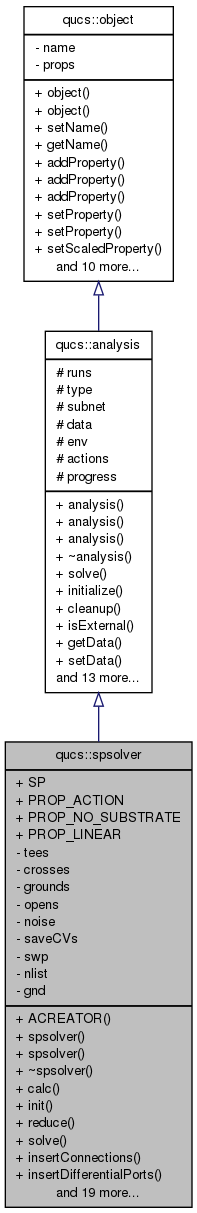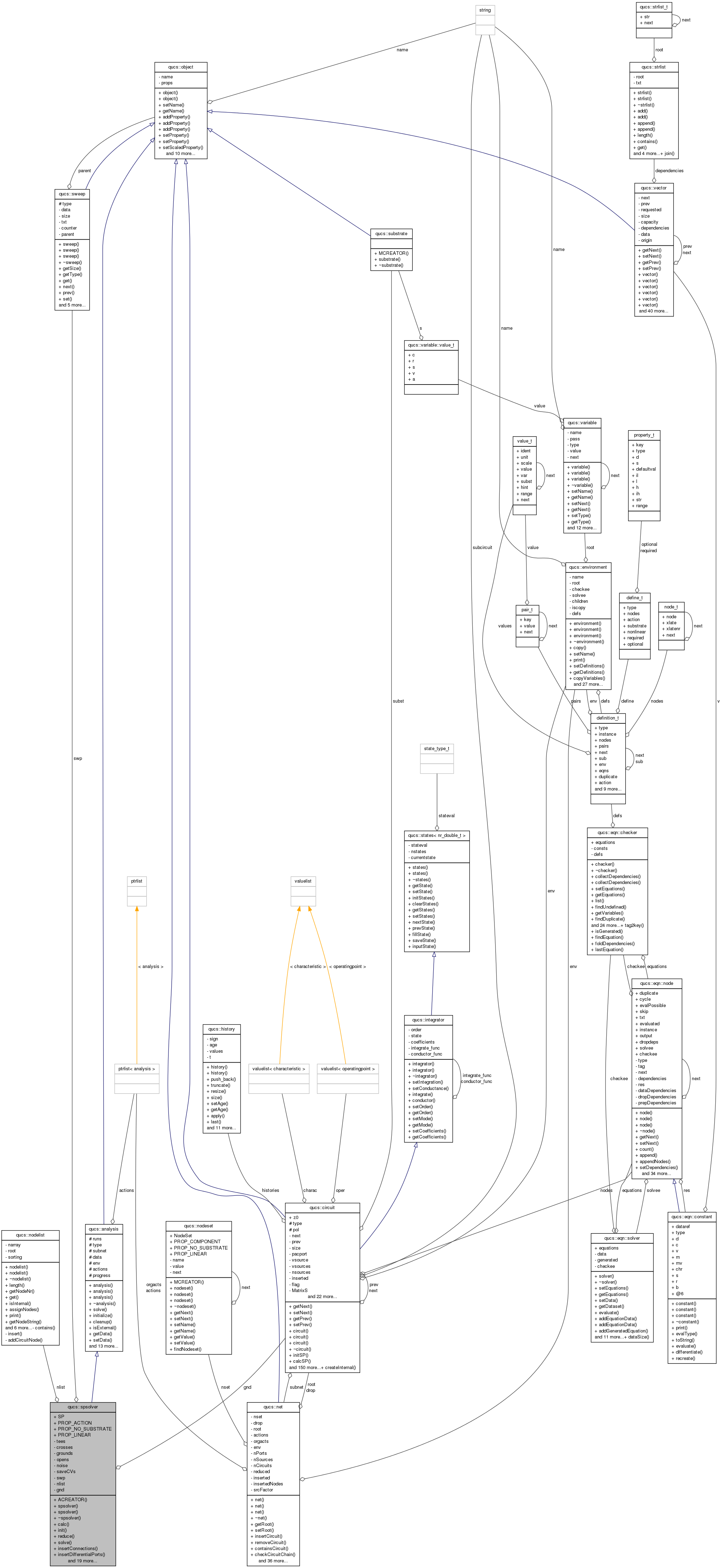Qucs-core  0.0.19
qucs::spsolver Class Reference

`#include <spsolver.h>`

Inheritance diagram for qucs::spsolver:[legend]
Collaboration diagram for qucs::spsolver:[legend]

## Public Member Functions

ACREATOR (spsolver)
spsolver (char *)
spsolver (spsolver &)
~spsolver ()
void calc (nr_double_t)
void init (void)
void reduce (void)
int solve (void)
placehoder for solution function
void insertConnections (void)
void insertDifferentialPorts (void)
void insertTee (node **, const char *)
void insertCross (node **, const char *)
void insertConnectors (node *)
void insertOpen (node *)
void insertGround (node *)
circuitinterconnectJoin (node *, node *)
circuitconnectedJoin (node *, node *)
void noiseConnect (circuit *, node *, node *)
void noiseInterconnect (circuit *, node *, node *)
void saveResults (nr_double_t)
void saveNoiseResults (nr_complex_t, nr_complex_t, nr_double_t, vector *)
char * createSP (int, int)
const char * createCV (const std::string &c, const std::string &n)
void saveCharacteristics (nr_double_t)
void dropTee (circuit *)
void dropCross (circuit *)
void dropOpen (circuit *)
void dropGround (circuit *)
void dropDifferentialPort (circuit *)
void dropConnections (void)

## Data Fields

SP
PROP_ACTION
PROP_NO_SUBSTRATE
PROP_LINEAR

int tees
int crosses
int grounds
int opens
int noise
int saveCVs
sweepswp
nodelistnlist
circuitgnd

## Detailed Description

Definition at line 1119 of file spsolver.cpp.

## Constructor & Destructor Documentation

 qucs::spsolver::spsolver ( char * n )

Definition at line 78 of file spsolver.cpp.

 qucs::spsolver::spsolver ( spsolver & n )

Definition at line 96 of file spsolver.cpp.

 qucs::spsolver::~spsolver ( )

Definition at line 89 of file spsolver.cpp.

## Member Function Documentation

 qucs::spsolver::ACREATOR ( spsolver )
 void qucs::spsolver::calc ( nr_double_t freq )

Definition at line 449 of file spsolver.cpp.

 circuit * qucs::spsolver::connectedJoin ( node * n1, node * n2 )

Definition at line 172 of file spsolver.cpp.

 const char * qucs::spsolver::createCV ( const std::string & c, const std::string & n )

Definition at line 1081 of file spsolver.cpp.

 char * qucs::spsolver::createSP ( int i, int j )

Definition at line 1075 of file spsolver.cpp.

 void qucs::spsolver::dropConnections ( void )

Definition at line 873 of file spsolver.cpp.

 void qucs::spsolver::dropCross ( circuit * c )

Definition at line 756 of file spsolver.cpp.

 void qucs::spsolver::dropDifferentialPort ( circuit * c )

Definition at line 955 of file spsolver.cpp.

 void qucs::spsolver::dropGround ( circuit * c )

Definition at line 808 of file spsolver.cpp.

 void qucs::spsolver::dropOpen ( circuit * c )

Definition at line 783 of file spsolver.cpp.

 void qucs::spsolver::dropTee ( circuit * c )

Definition at line 743 of file spsolver.cpp.

 void qucs::spsolver::init ( void )

Definition at line 556 of file spsolver.cpp.

 void qucs::spsolver::insertConnections ( void )

Definition at line 820 of file spsolver.cpp.

 void qucs::spsolver::insertConnectors ( node * n )

Definition at line 635 of file spsolver.cpp.

 void qucs::spsolver::insertCross ( node ** nodes, const char * name )

Definition at line 713 of file spsolver.cpp.

 void qucs::spsolver::insertDifferentialPorts ( void )

Definition at line 919 of file spsolver.cpp.

 void qucs::spsolver::insertGround ( node * n )

Definition at line 792 of file spsolver.cpp.

 void qucs::spsolver::insertOpen ( node * n )

Definition at line 770 of file spsolver.cpp.

 void qucs::spsolver::insertTee ( node ** nodes, const char * name )

Definition at line 686 of file spsolver.cpp.

 circuit * qucs::spsolver::interconnectJoin ( node * n1, node * n2 )

Definition at line 110 of file spsolver.cpp.

 void qucs::spsolver::noiseConnect ( circuit * result, node * n1, node * n2 )

Definition at line 344 of file spsolver.cpp.

 void qucs::spsolver::noiseInterconnect ( circuit * result, node * n1, node * n2 )

Definition at line 277 of file spsolver.cpp.

 void qucs::spsolver::reduce ( void )

Definition at line 460 of file spsolver.cpp.

 void qucs::spsolver::saveCharacteristics ( nr_double_t freq )

Definition at line 1088 of file spsolver.cpp.

 void qucs::spsolver::saveNoiseResults ( nr_complex_t s, nr_complex_t c, nr_double_t z0, vector * f )

Definition at line 1038 of file spsolver.cpp.

 void qucs::spsolver::saveResults ( nr_double_t freq )

Definition at line 970 of file spsolver.cpp.

 int qucs::spsolver::solve ( void ) ` [virtual]`

placehoder for solution function

Virtual solution function intended to be overridden by the inheiriting class's solution function.

Reimplemented from qucs::analysis.

Definition at line 567 of file spsolver.cpp.

## Field Documentation

 int qucs::spsolver::crosses` [private]`

Definition at line 75 of file spsolver.h.

 circuit* qucs::spsolver::gnd` [private]`

Definition at line 80 of file spsolver.h.

 int qucs::spsolver::grounds` [private]`

Definition at line 75 of file spsolver.h.

 nodelist* qucs::spsolver::nlist` [private]`

Definition at line 79 of file spsolver.h.

 int qucs::spsolver::noise` [private]`

Definition at line 76 of file spsolver.h.

 int qucs::spsolver::opens` [private]`

Definition at line 75 of file spsolver.h.

Definition at line 1120 of file spsolver.cpp.

Definition at line 1120 of file spsolver.cpp.

Definition at line 1120 of file spsolver.cpp.

 int qucs::spsolver::saveCVs` [private]`

Definition at line 77 of file spsolver.h.

 qucs::spsolver::SP

Definition at line 1120 of file spsolver.cpp.

 sweep* qucs::spsolver::swp` [private]`

Definition at line 78 of file spsolver.h.

 int qucs::spsolver::tees` [private]`

Definition at line 75 of file spsolver.h.

The documentation for this class was generated from the following files: